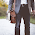## HOW TO SOLVE LINEAR EQUATIONS OF THREE VARIABLES BY MATRIX METHOD

How to find solutions of system of linear equations of three variables with  the help of Matrix Method. Let us solve three linear equations of three variables given below by Matrix Method.

These are not simple linear equations to solve. But these equations can be made simple by following substitution .

After substitution , these equations are reduced to following simple linear equations
2x + 3y + 4z = 3
3x - 4y + 5z = 5
x + 2y - 3z = 6
To solve these equations by Matrix Method , Let us transform theses set of linear equations to Matrix form

AX = B,   and  =A-1 B---------------- (4)

where A is matrix comprises the coefficients  x , y and z respectively as shown in the matrix given below , And B is the matrix consist of constant terms on right hand side of these equations and X is the matrix of variables in the linear equations.

1st of all we have to find A- and then product of the matrices A-1 and  as mentioned in equation (4). But to find inverse of matrix A, its determinant value must be non zero   ( Note it) .
Let us find determinant value of Matrix A as follows :-

|A| = 2[(-4)(-3)–(5)(2)]-3[(3)(-3)-(5)(1)]+4[(3)(2)-(-4)(1)]

|A| = 2[12-10]-3[-9-5]+4[6+4]
|A| = 2 -3[-14]+4
|A| = 4 +42+40
|A| = 86
Since the value of Determinant of Matrix a is non zero.
⇒    A-1  exists .
To find inverse ,we have to find ad joint of Matrix A . And to find Ad joint of any Matrix , we have to find Co factor Matrix .

## What is Co Factor Matrix

Co factor Matrix can be obtained by the co factors of all the elements of Matrix written in same place  where the element was written originally.
Formula for Co factor of any element
Cij = (-1)i+jMij

### Co factor of A11

Co factor of A11   element can be calculated by eliminating 1st row and 1st column and solving the remaining determinant.
(-1)1+1M11
= 12 - 10 = 2

### Co factor of A12

Co factor of A12   element can be calculated   by eliminating 1st row and 2nd column and solving the remaining determinant.
(-1)1+2M12

= -1(-9 - 5 )= 14

### Co factor of A13

Co factor of A13   element  can be  calculated   by eliminating 1st row and 3rd column and solving the remaining determinant.
(-1)1+3M13
= 6 + 4 = 10

### Co factor of A21

Co factor of A21   element can be calculated   by eliminating 2nd row and 1st column and solving the remaining determinant.
(-1)2+1M11

= -1(- 9 - 8) = 17

### Co factor of A22

Co factor of A22   element  can be calculated   by eliminating 2nd row and 2nd column and solving the remaining determinant.
(-1)2+2M22
= - 6 - 4 = -10

### Co factor of A23

Co factor of A23   element can be calculated   by eliminating 2nd row and 3rd column and solving the remaining determinant.

(-1)2+3M23
= -1(4 - 3) = -1

### Co factor of A31

Co factor of A31   element can be calculated   by eliminating 3rd row and 1st column and solving the remaining determinant.

(-1)3+1M31
= 15 + 16 = 31

### Co factor of A32

Co factor of A32   element  can  be calculated   by eliminating 3rd row and 2nd column and solving the remaining determinant.

(-1)3+2M32
= -1(10 - 12) = 2

### Co factor of A33

Co factor of A33   element  can  be calculated   by eliminating 3rd row and 3rd column and solving the remaining determinant.

(-1)3+3M33
= - 8 - 9 = -17

## Co Factor Matrix of A

Now write all the co factors calculated in Matrix Form as follows

## Ad joint Matrix of A

To find the Ad Joint of  Co factor Matrix, transform 1st row to 1st column, 2nd row to 2nd column  and 3rd rows to 3rd column as follows

## Formula to Find   A-1

To Find the value of  A-1 ,Putting the value of Adj A in the formula given below.

## Find values of x ,y and z

Multiplying both the matrices which are on the right side.
Simplifying the matrix so obtained
Dividing each element by 86 to get matrix of 3×1 (i. e. Perform scalar multiplication of matrix ).

Using the equality of two matrices .We can write the values of x, y  and z respectively like this

x =277/86  , y = 4/86  and z = -77/86

Now the values of P, Q and R can be calculated by putting the values of x , y and z in equations (1) , (2 ) and (3) respectively.

P = 86/277  ,Q = 86/4   and R = -86/77

Don't Forget to Watch this Video of same Method

## Verification of solution

Putting values of P , Q and R in given  equations , these values must satisfies  three given equations
From 1st equation

Similarly other two equations can be verified

TO CRACK  ANY COMPETITIVE EXAM VISIT THIS YOUTUBE CHANNEL FOR MATHS AND REASONING

## EXAM CRACKER## My Previous Posts

Don't forget to   read this posts

Quiz of  Mathematics For You

## Conclusion

I have discussed the method of solving the System of linear Equations with the help of Matrix Method , method to find inverse of a matrix ,How to find inverse of 3×3 Matrix,how to solve determinant,how to solve system of equation by matrix,matrix method of solving system of equations of three variables,If you liked the post Don't  forget to share it with your friends , And in case of any improvement please make use of Comment Box .

## Appeal

If you are a mathematician Don't forget to visit my Mathematics You tube channel , Mathematics Website and Mathematics Facebook Page , whose links are given below

Maths Website , Facebook Page and You Tube Channel

Share:

1.Thanks for sharing this topic.

2.Matrix method is my favorite topic in Mathematics because it is interesting to solve these type of equations. Because of Mathematics is my favorite subject I always do searching for Mathematics topics online and mostly I learned Mathematics easy tricks from https://smiletutor.sg/maths-tuition/. Matrix method is very scoring topic and main reason my good rank in Mathematics is this topic.

3.4.